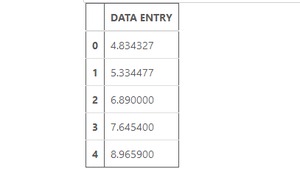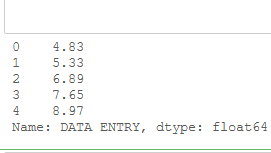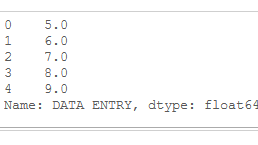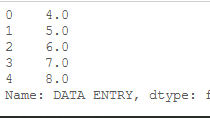Related Articles

# Methods to Round Values in Pandas DataFrame

• Last Updated : 18 Aug, 2020

There are various ways to Round Values in Pandas DataFrame so let’s see each one by one:

Let’s create a Dataframe with ‘Data Entry’ Column only:

Code:

## Python3

 `# import Dataframe class``# from pandas library``from` `pandas ``import` `DataFrame`` ` `# import numpy library``import` `numpy as np`` ` `# dictionary``Myvalue ``=` `{``'DATA ENTRY'``: [``4.834327``, ``5.334477``,``                          ``6.89``, ``7.6454``, ``8.9659``]} `` ` `# create a Dataframe``df ``=` `DataFrame(Myvalue,``               ``columns ``=` `[``'DATA ENTRY'``])`` ` `# show the dataframe``df`

Output:Method 1:  Using numpy.round().

Syntax: numpy.round_(arr, decimals = 0, out = None)

Return: An array with all array elements being
rounded off, having same type as input.

This method can be  used to round value to specific decimal places for any particular column or can also be used to round the value of the entire data frame to the specific number of decimal places.

Example: Rounding off the value of  the “DATA ENTRY” column up to 2 decimal places.

## Python3

 `# import Dataframe class``# from pandas library``from` `pandas ``import` `DataFrame`` ` `# import numpy library``import` `numpy as np`` ` `# dictionary``Myvalue ``=` `{``'DATA ENTRY'``: [``4.834327``, ``5.334477``,``                          ``6.89``, ``7.6454``, ``8.9659``]} `` ` `# create a Dataframe``df ``=` `DataFrame(Myvalue,``               ``columns ``=` `[``'DATA ENTRY'``])`` ` `# Rounding value of 'DATA ENTRY' ``# column upto 2 decimal places``roundplaces ``=` `np.``round``(df[``'DATA ENTRY'``],``                       ``decimals ``=` `2``) `` ` `# show the rounded value``roundplaces`

Output:Method 2: Using Dataframe.apply() and numpy.ceil() together.

Syntax: Dataframe/Series.apply(func, convert_dtype=True, args=())

Return: Pandas Series after applied function/operation.

Syntax: numpy.ceil(x[, out]) = ufunc ‘ceil’)

Return: An array with the ceil of each element of float data-type.

These methods are used to round values to ceiling value(smallest integer value greater than particular value).

Example: Rounding off the value of a particular column.

## Python3

 `# import Dataframe from ``# pandas library``from` `pandas ``import` `DataFrame`` ` `# import numpy``import` `numpy as np`` ` `# dictionary``Myvalue ``=` `{``'DATA ENTRY'``: [``4.834327``, ``5.334477``,``                          ``6.89``, ``7.6454``, ``8.9659``]} `` ` `# create a Dataframe``df ``=` `DataFrame(Myvalue, ``               ``columns ``=` `[``'DATA ENTRY'``])`` ` `# Here we are rounding the ``# value to its ceiling values``roundUp ``=` `df[``'DATA ENTRY'``].``apply``(np.ceil) `` ` `# show the rounded value``roundUp`

Output:Method 3: Using Dataframe.apply() and numpy.floor() together.

Syntax: numpy.floor(x[, out]) = ufunc ‘floor’)

Return: An array with the floor of each element.

These methods are used to round values to floor value(largest integer value smaller than particular value).

Example: Rounding off the value of the “DATA ENTRY” column to its corresponding Floor value.

## Python3

 `# import Dataframe class ``# from pandas library``from` `pandas ``import` `DataFrame`` ` `# import numpy library``import` `numpy as np`` ` `# dictionary``Myvalue ``=` `{``'DATA ENTRY'``:[``4.834327``, ``5.334477``, ``                         ``6.89``, ``7.6454``, ``8.9659``] } ``# create a Dataframe``df ``=` `DataFrame(Myvalue, ``               ``columns ``=` `[``'DATA ENTRY'``]) `` ` `# Rounding of Value to its Floor value ``rounddown ``=` `df[``'DATA ENTRY'``].``apply``(np.floor)  `` ` `# show the rounded value``rounddown`

Output:Attention geek! Strengthen your foundations with the Python Programming Foundation Course and learn the basics.

To begin with, your interview preparations Enhance your Data Structures concepts with the Python DS Course. And to begin with your Machine Learning Journey, join the Machine Learning – Basic Level Course

My Personal Notes arrow_drop_up